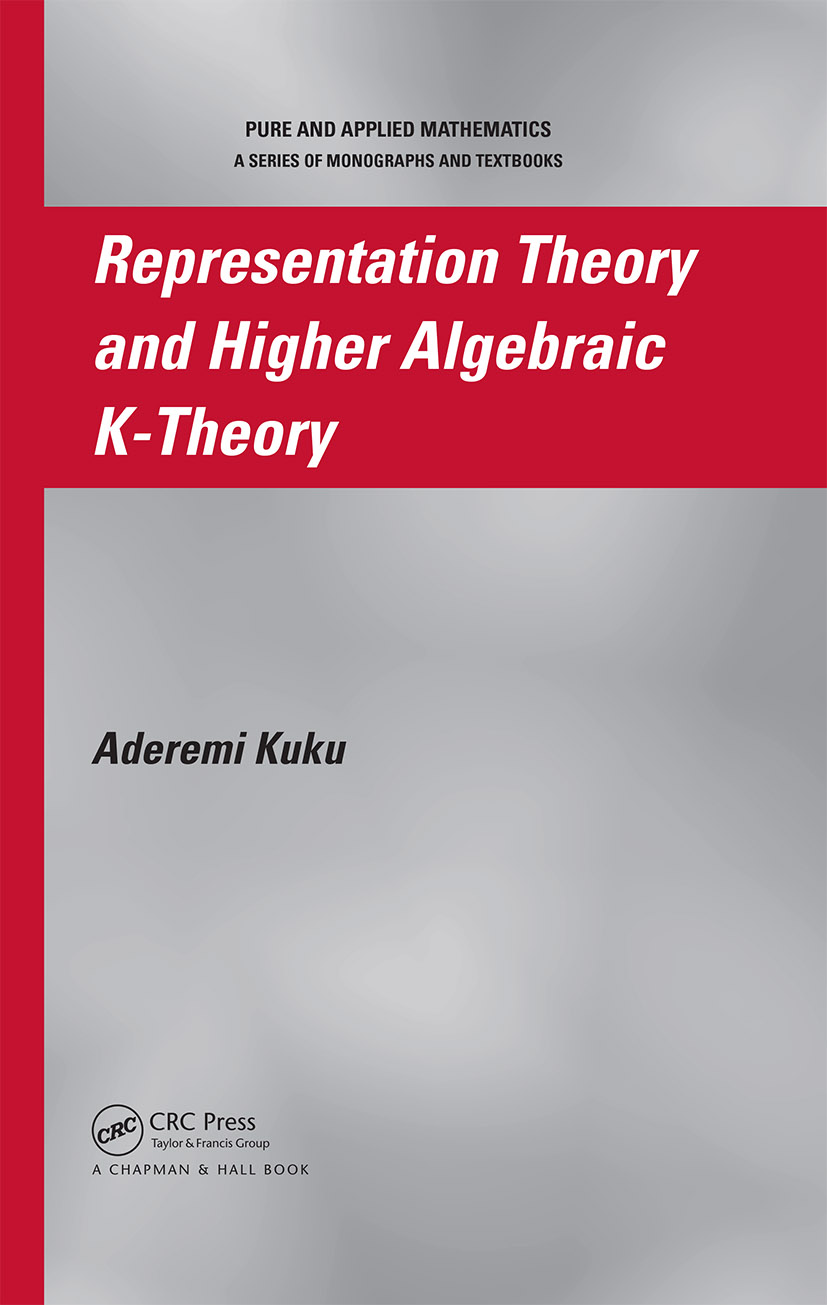# Representation Theory and Higher Algebraic K-Theory

## 1st Edition

Chapman and Hall/CRC

442 pages

##### Purchasing Options:\$ = USD
Hardback: 9781584886037
pub: 2006-09-27
\$145.00
x
eBook (VitalSource) : 9780429148552
pub: 2016-04-19
from \$28.98

FREE Standard Shipping!

### Description

Representation Theory and Higher Algebraic K-Theory is the first book to present higher algebraic K-theory of orders and group rings as well as characterize higher algebraic K-theory as Mackey functors that lead to equivariant higher algebraic K-theory and their relative generalizations. Thus, this book makes computations of higher K-theory of group rings more accessible and provides novel techniques for the computations of higher K-theory of finite and some infinite groups.

Authored by a premier authority in the field, the book begins with a careful review of classical K-theory, including clear definitions, examples, and important classical results. Emphasizing the practical value of the usually abstract topological constructions, the author systematically discusses higher algebraic K-theory of exact, symmetric monoidal, and Waldhausen categories with applications to orders and group rings and proves numerous results. He also defines profinite higher K- and G-theory of exact categories, orders, and group rings. Providing new insights into classical results and opening avenues for further applications, the book then uses representation-theoretic techniques-especially induction theory-to examine equivariant higher algebraic K-theory, their relative generalizations, and equivariant homology theories for discrete group actions. The final chapter unifies Farrell and Baum-Connes isomorphism conjectures through Davis-Lück assembly maps.

### Reviews

"This book provides a unified treatment of deep results … Written by a leading expert in the field, this monograph will be indispensable for anyone interested in contemporary representation theory and its K-theoretic aspects."

-EMS Newsletter, June 2007

"This book is a unique contribution to the literature. It surveys a number of important topics in algebraic K-theory, the theory of group rings, and equivariant topology which up until now have been widely scattered in the literature and have not been readily available in book form."

– Jonathan M. Rosenberg, in Mathematical Reviews, 2007k

Introduction

REVIEW OF CLASSICAL ALGEBRAIC K-THEORY AND REPRESENTAION THEORY

Notes on Notations

Category of Representations and Constructions of Grothendieck Groups and Rings

Category of representations and G-equivariant categories

Grothendieck group associated with a semi-group

K0 of symmetric monoidal categories

K0 of exact categories - definitions and examples

Exercises

Some Fundamental Results on K0 of Exact and Abelian Categories with Applications to Orders and Group Rings

Some fundamental results on K0 of exact and Abelian categories

Some finiteness results on K0 and G0 of orders and groupings

Class groups of Dedekind domains, orders, and group rings plus some applications

Decomposition of G0 (RG) (G Abelian group) and extensions to some non-Abelian groups

Exercises

K1, K2 of Orders and Group Rings

Definitions and basic properties

K1, SK1 of orders and group-rings; Whitehead torsion

The functor K2

Exercises

Some Exact Sequences; Negative K-Theory

Mayer-Vietoris sequences

Localization sequences

Exact sequence associated to an ideal of a ring

Negative K-theory K-n, n positive integer

Lower K-theory of group rings of virtually infinite cyclic groups

HIGHER ALGEBRAIC K-THEORY AND INTEGRAL REPRESENTATIONS

Higher Algebraic K-Theory-Definitions, Constructions, and

Relevant Examples

The plus construction and higher K-theory of rings

Classifying spaces and higher K-theory of exact categories-constructions and examples

Higher K-theory of symmetric monoidal categories-definitions and examples

Higher K-theory of Waldhausen categories-definitions and examples

Exercises

Some Fundamental Results and Exact Sequences in Higher K-Theory

Some fundamental theorems

Localization

Fundamental theorem of higher K-theory

Some exact sequences in the K-theory of Waldhausen categories

Exact sequence associated to an ideal, excision, and Mayer-Vietoris sequences

Exercises

Some Results on Higher K-Theory of Orders, Group Rings and

Modules over "EI" Categories

Some finiteness results on Kn, Gn, SKn, SGn of orders and groupings

Ranks of Kn(?), Gn(?) of orders and group rings plus some consequences

Decomposition of Gn(RG) n = 0, G finite Abelian group;

Extensions to some non-Abelian groups, e.g., quaternion and dihedral groups

Higher dimensional class groups of orders and group rings

Higher K-theory of group rings of virtually infinite cyclic groups

Higher K-theory of modules over "EI" -categories

Higher K-theory of P(A)G, A maximal orders in division algebras, G finite group

Exercises

Mod-m and Profinite Higher K-Theory of Exact Categories, Orders, and Groupings

Mod-m K-theory of exact categories, rings and orders

Profinite K-theory of exact categories, rings and orders

Profinite K-theory of p-adic orders and semi-simple algebras

Continuous K-theory of p-adic orders

MACKEY FUNCTORS, EQUIVARIANT HIGHER ALGEBRAIC K-THEORY, AND EQUIVARIANT HOMOLOGY THEORIES

Exercises

Mackey, Green, and Burnside Functors

Mackey functors

Cohomology of Mackey functors

Green functors, modules, algebras, and induction theorems

Based category and the Burnside functor

Induction theorems for Mackey and Green functors

Defect basis of Mackey and Green functors

Defect basis for KG0 -functors

Exercises

Equivariant Higher Algebraic K-Theory Together with Relative

Generalizations for Finite Group Actions

Equivariant higher algebraic K-theory

Relative equivariant higher algebraic K-theory

Interpretation in terms of group rings

Some applications

Exercises

Equivariant Higher K-Theory for Profinite Group Actions

Equivariant higher K-theory (absolute and relative)

Cohomology of Mackey functors (for profinite groups)

Exercises

Equivariant Higher K-Theory for Compact Lie Group Actions

Mackey and Green functors on the category A(G) of homogeneous spaces

An equivariant higher K-theory for G-actions

Induction theory for equivariant higher K-functors

Exercise

Equivariant Higher K-Theory for Waldhausen Categories

Equivariant Waldhausen categories

Equivariant higher K-theory constructions for Waldhausen categories

Applications to complicial bi-Waldhausen categories

Applications to higher K-theory of group rings

Exercise

Equivariant Homology Theories and Higher K-Theory of Group Rings

Classifying space for families and equivariant homology theory

Assembly maps and isomorphism conjectures

Farrell-Jones conjecture for algebraic K-theory

Baum-Connes conjecture

Davis-Lück assembly map for BC conjecture and its identification with analytic assembly map

Exercise

Appendices

A: Some computations

B: Some open problems

References

Index

### Subject Categories

##### BISAC Subject Codes/Headings:
MAT002000
MATHEMATICS / Algebra / General
MAT012000
MATHEMATICS / Geometry / General
MAT022000
MATHEMATICS / Number Theory